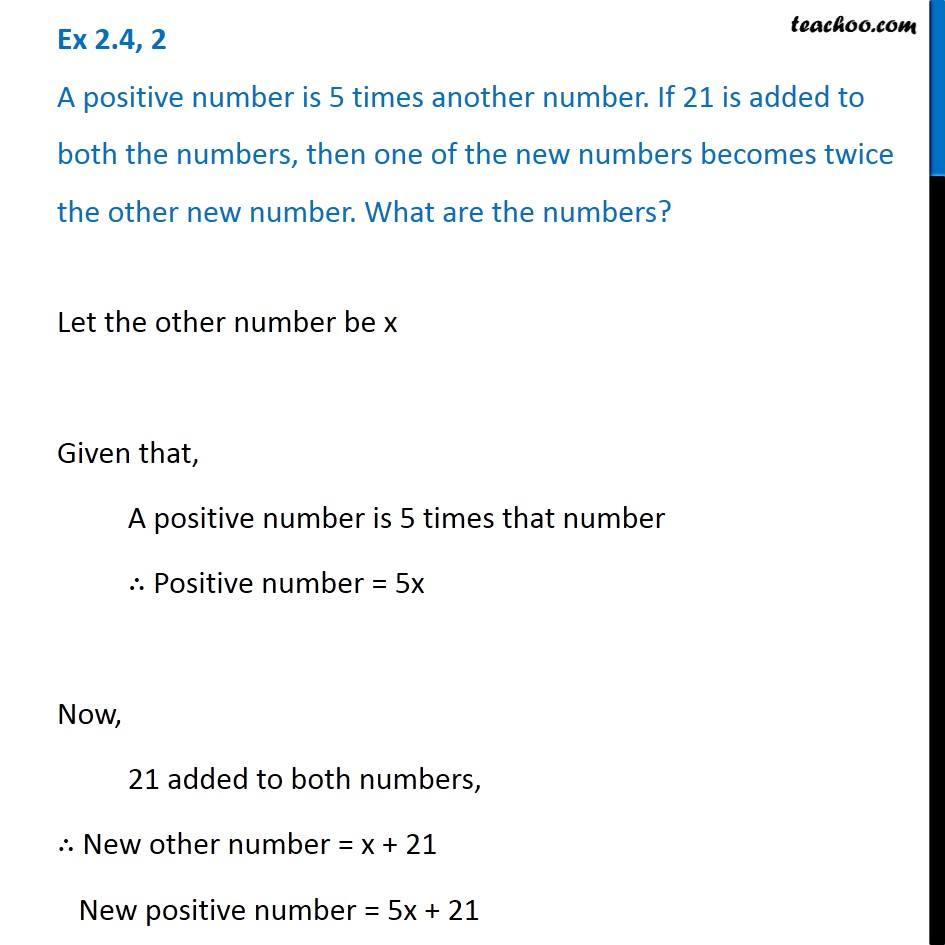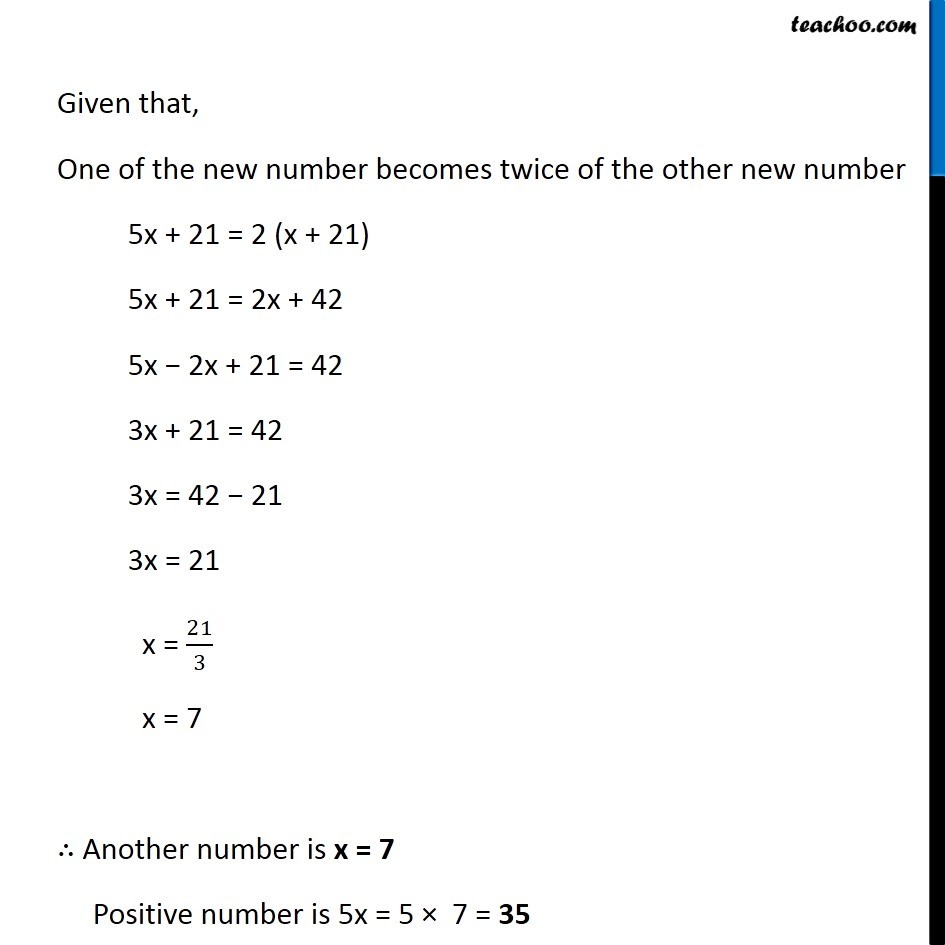Solving difficult equations

Chapter 2 Class 8 Linear Equations in One Variable
Serial order wiseLearn in your speed, with individual attention - Teachoo Maths 1-on-1 Class

### Transcript

Question 2 A positive number is 5 times another number. If 21 is added to both the numbers, then one of the new numbers becomes twice the other new number. What are the numbers?Let the other number be x Given that, A positive number is 5 times that number ∴ Positive number = 5x Now, 21 added to both numbers, ∴ New other number = x + 21 New positive number = 5x + 21 Given that, One of the new number becomes twice of the other new number 5x + 21 = 2 (x + 21) 5x + 21 = 2x + 42 5x − 2x + 21 = 42 3x + 21 = 42 3x = 42 − 21 3x = 21 x = 21/3 x = 7 ∴ Another number is x = 7 Positive number is 5x = 5 × 7 = 35 Rough Here, First new number = x + 21 Second number = 5x + 21 So, second number is greater Second number will be twice of first ∴ It will be 5x + 21 = 2 (x + 21)